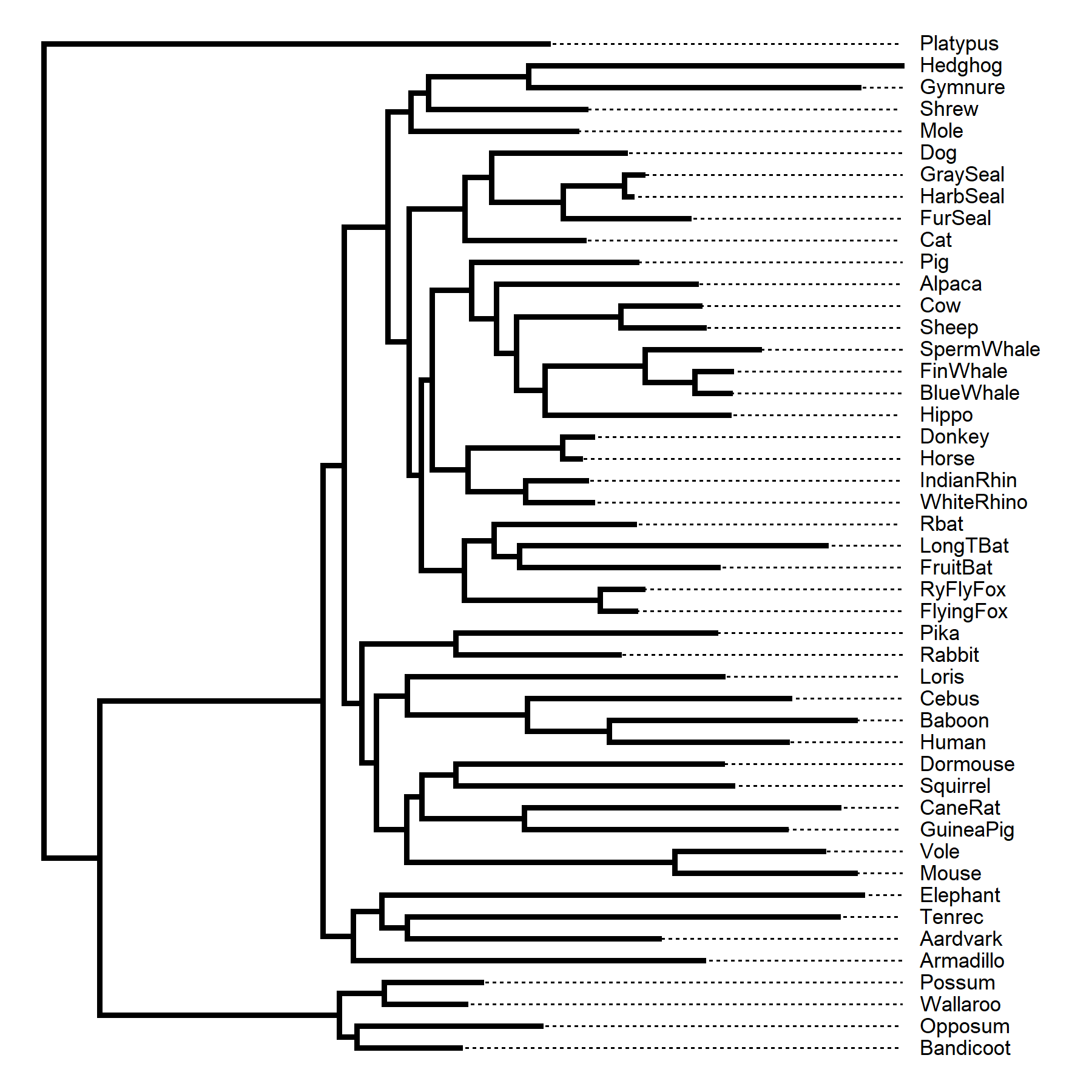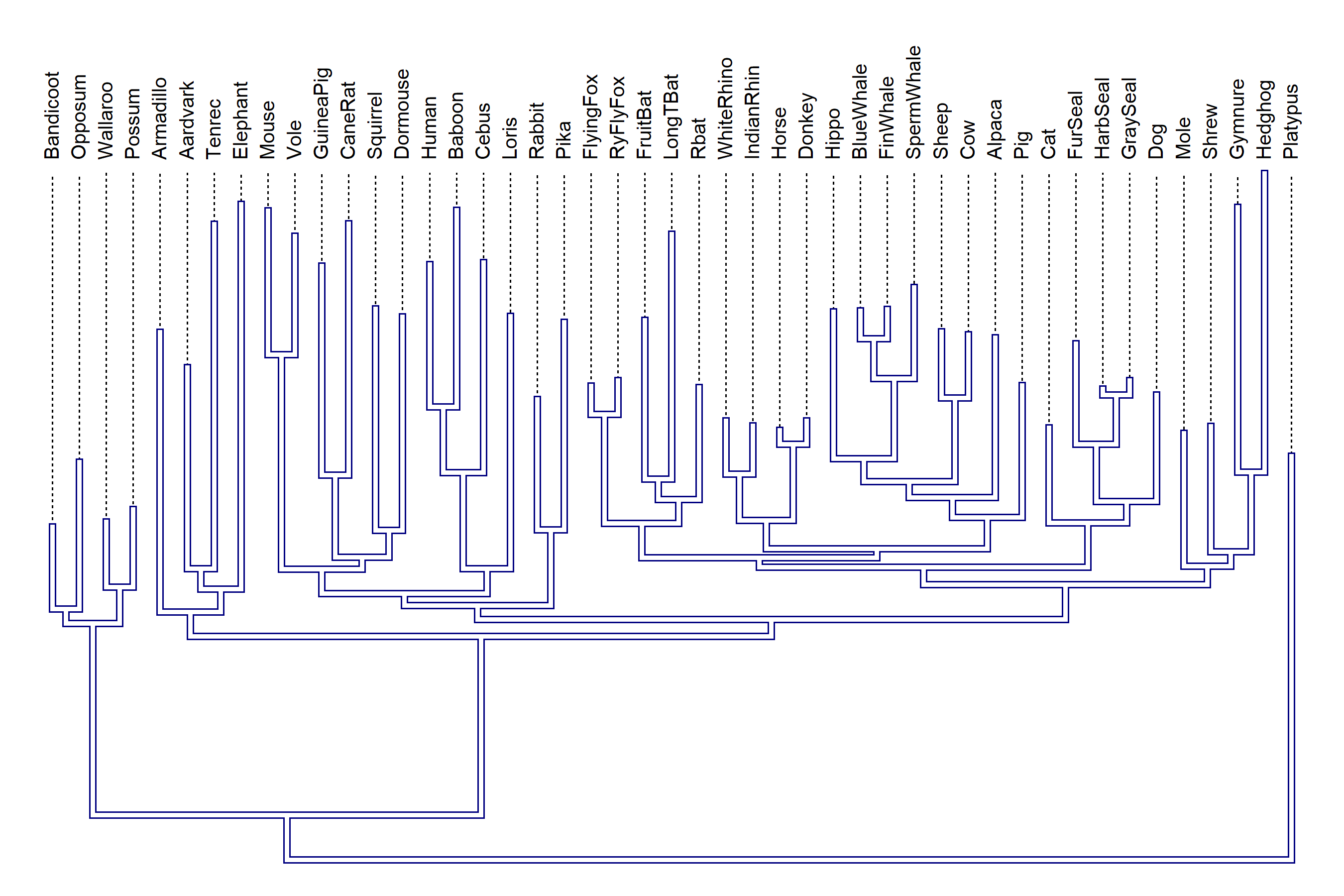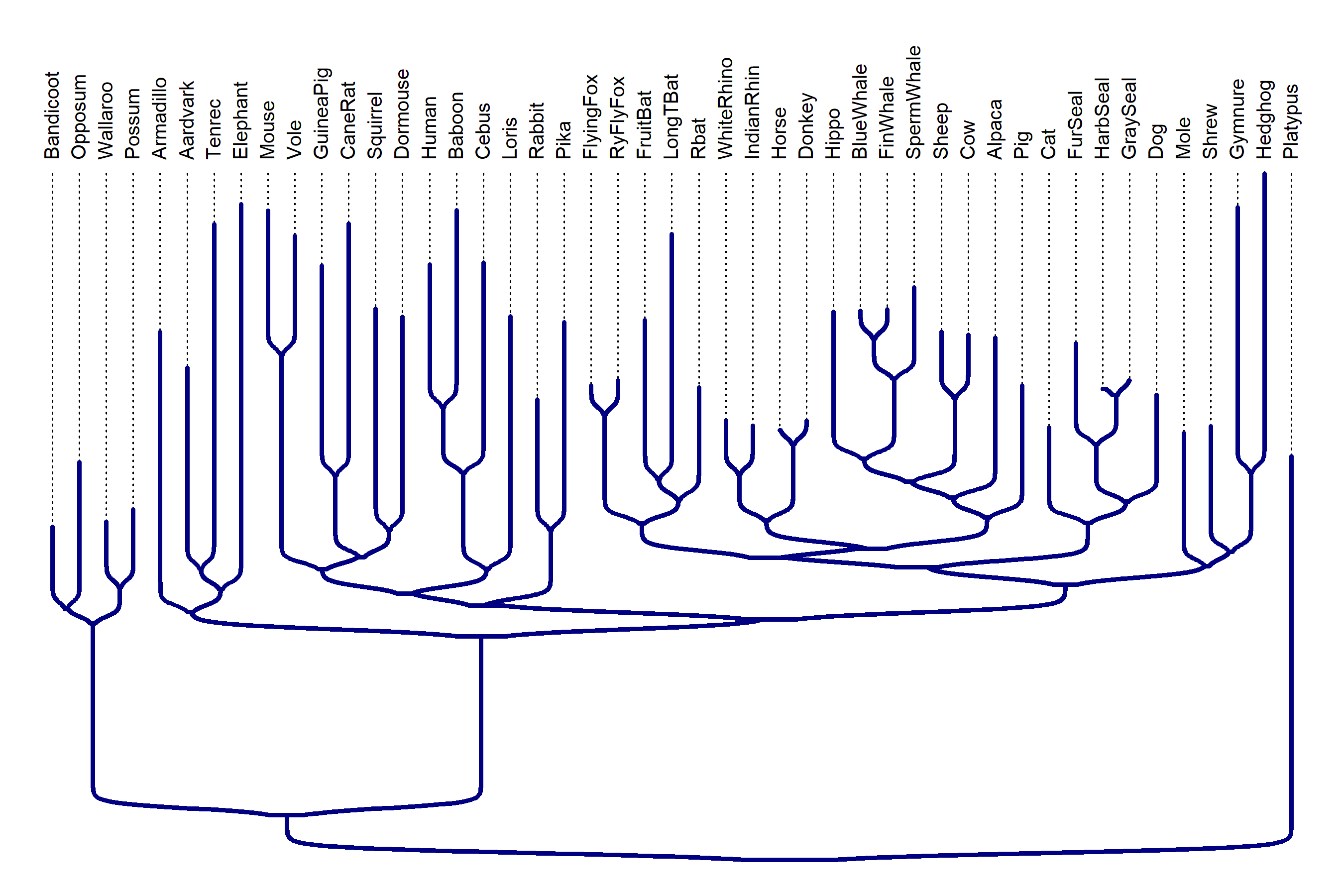## Tuesday, November 7, 2023

### Plotting trick to connect aligned labels to the tips of a graphed non-ultrametric tree using phytools

I feel certain that I’ve shown this before, but the following is a simple ‘hack’ to plot a non-ultrametric rooted tree with aligned tip labels & dotted lines connecting each tip to the corresponding label.

To illustrate it, I’m going to quickly estimate an empirical tree using Maximum Likelihood with the excellent package phangorn.

``````library(phytools)
library(phangorn)
data("Laurasiatherian")
mammal.ML<-pml_bb(Laurasiatherian,model="GTR+G(4)+I",
control=pml.control(trace=0))
mammal.ML
``````
``````## model: GTR+G(4)+I
## loglikelihood: -44565.74
## unconstrained loglikelihood: -17300.92
## Proportion of invariant sites: 0.292363
## Model of rate heterogeneity: Discrete gamma model
## Number of rate categories: 4
## Shape parameter: 0.5958122
##         Rate Proportion
## 1 0.00000000  0.2923630
## 2 0.07383092  0.1769092
## 3 0.43798783  0.1769092
## 4 1.24239881  0.1769092
## 5 3.89839853  0.1769092
##
## Rate matrix:
##           a          c          g         t
## a  0.000000  3.6283208 14.0268552  3.887259
## c  3.628321  0.0000000  0.4366659 25.447966
## g 14.026855  0.4366659  0.0000000  1.000000
## t  3.887259 25.4479659  1.0000000  0.000000
##
## Base frequencies:
##         a         c         g         t
## 0.3321866 0.1990791 0.2040652 0.2646691
``````

This dataset has a very clear outgroup, the platypus, so I’ll use that to root it as follows. Just for aesthetic purposes, I’ll root the tree 10% of the way along the edge leading to “Platypus” rather than at the node itself (but this is non-scientific – we don’t have any information about the real position of the root along the edge in this tree).

``````mammal_tree<-mammal.ML\$tree
nn<-which(mammal_tree\$tip.label=="Platypus")
mammal_tree<-reroot(mammal_tree,nn,
position=0.1*mammal_tree\$edge.length[which(mammal_tree\$edge[,2]==nn)])
mammal_tree
``````
``````##
## Phylogenetic tree with 47 tips and 46 internal nodes.
##
## Tip labels:
##   Platypus, Wallaroo, Possum, Bandicoot, Opposum, Armadillo, ...
## Node labels:
##   Root, 1, 0.999, 0.885, 1, 0.89, ...
##
## Rooted; includes branch lengths.
``````

Now I’m ready for my plotting routine.

``````par(lty="dotted")
plotTree(force.ultrametric(mammal_tree,method="extend",message=FALSE),
lwd=1,fsize=0.7,offset=0.5)
xlim<-get("last_plot.phylo",envir=.PlotPhyloEnv)\$x.lim
par(lty="solid")
``````The way this code chunk works is that I first plot my tree but with all tips extended to line up with a total length equal to the highest tip in the phylogeny. (This is accomplished by internally calling `phytools::force.ultrametric`.) Next, I pull out the x-axis limits using `get("last_plot.phylo",envir=.PlotPhyloEnv)`. Finally, I overplot the ultrametric tree with my estimated phylogeny.

Having done this, it should be fairly evident that we can apply it to other plotting styles & cases as well. In the example below, I plot my tree upwards & also overplot in white to create a “outline” effect. Because I’m plotting upwards, I need to switch `ylim` for `xlim`.

``````par(lty="dotted")
plotTree(force.ultrametric(mammal_tree,method="extend",
message=FALSE),lwd=1,fsize=0.8,offset=1,direction="upwards")
ylim<-get("last_plot.phylo",envir=.PlotPhyloEnv)\$y.lim
par(lty="solid")
direction="upwards",color="#000080")
direction="upwards",color="white")
``````Unfortunately, the same approach didn’t work for `phytools::sigmoidPhylogram`, so I thought of this alternative hack.

``````plotTree(force.ultrametric(mammal_tree,method="extend",
message=FALSE),lwd=1,fsize=0.8,offset=1,direction="upwards",
color="transparent")
pp.1<-get("last_plot.phylo",envir=.PlotPhyloEnv)
plotTree(mammal_tree,lwd=1,fsize=0.8,offset=1,direction="upwards",
pp.2<-get("last_plot.phylo",envir=.PlotPhyloEnv)
for(i in 1:Ntip(mammal_tree))
lines(rep(pp.1\$xx[i],2),c(pp.1\$yy[i],pp.2\$yy[i]),lty="dotted")